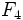# Field:F4

## Definition

This field, denoted$F_4$ or$GF(4)$, is the unique field (up to isomorphism) with four elements. It can be defined as:$F_2[x]/(x^2 + x + 1)$.

## Related groups

Group functor Value GAP ID